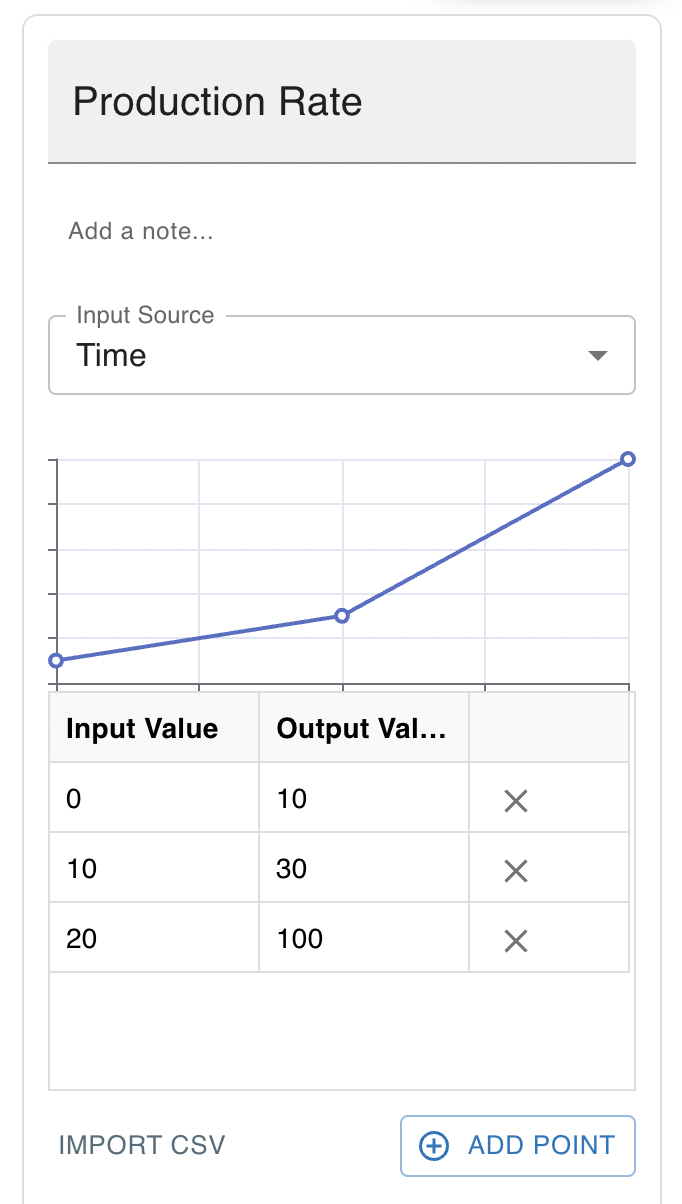# Converters

Often you will want to add structured data or empirical data to your model. For instance, if you were modeling a lake, you might want your model to have access to historical precipitation data that was recorded periodically. Alternatively, you might want to know the actual, surveyed surface area of the lake, given the volume of the lake.

Insight Maker makes using this easy with its converter primitive (similar to graphical function) which allows you to create an input-output relationship or graph. When the input source to a converter primitive takes on an input value, the converter takes on the corresponding output value which may then be used by other primitives that reference the converter. The input can be the current time (in seconds, minutes, hours, etc…) or the value of some other primitive that is linked to the converter.

For converters, if you have not specified a specific output value for a given input, the outputs closest to the input will be combined to develop an appropriate output based on an interpolation method you have specified. For illustration, take the following example input-output table for a converter whose input source is the current time in years and where linear interpolation has been selected.At the start of the simulation (when the time is 0 years), the converter will take on the value of 0. Ten years into the simulation (when the time is 10 years), the converter will take on a value of 100.

You can enter data into the converter by hand or import a two-columned comma separated dataset (CSV file) that may be generated by Google Sheets, Microsoft Excel or other spreadsheet programs.